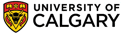﻿ Don't sum locantsBasic IUPAC Organic Nomenclature

Don't sum locants!

Simply put, there is NO IUPAC rule based on summing the locants despite what you may have been taught elsewhere!

However, to be honest, the sum idea works in most cases (unfortunately). But, learning the "sum rule" typically means one doesn't take the time to learn the first point of difference rule which is required in several other situations. Therefore, it is recommended that you learn the first point of difference rule as you will probably need to learn it at some point anyway, and it really isn't any more difficult to learn!

(summing a list of numbers versus comparing a list of numbers written in numerical order).

HINT:

• for each possible numbering scheme, list out the locants in numerical order
• compare the "lists" to locate the first point of difference, i.e. the first time you encounter a low number
• at the first point of difference, select the lower locant choice.

Here are some examples of the appli
cation of the first point of difference rule to generate the correct name where the "sum rule" fails:

Consider the following example (from an IUPAC nomenclature website):Longest chain is C10 First, look at numbering the longest chain from left to rightLocants would be 3,4,9 Second, look at numbering the longest chain from right to leftLocants would be 2,7,8

If we were to sum, then the total from the first numbering scheme (left to right) = 16, the second scheme (right to left) = 17 which means if the sum rule existed, it would make scheme 1 the answer.

However, let's follow a method for identifying the first point of difference... list out the locant set for each scenario in numerical order. Then compare the lists looking for the first time the numbers are different:

 list out the locant set for each scenario with the locants in numerical order compare the lists looking for the first time the numbers are different (mouseover) pick the scenario with the lower number at the first difference (here 2 is less than 3)The correct name is 2,7,8-trimethyldecaneConsider the following example (from Carey "Organic Chemistry" by McGraw-Hill):Longest chain is C8 First, look at numbering the longest chain from left to rightLocants would be 2,3,3,7,7 Second, look at numbering the longest chain from right to leftLocants would be 2,2,6,6,7

If we were to sum, then the total from the first numbering scheme (left to right) = 22, the second scheme (right to left) = 23 which means if the sum rule existed, it would make scheme 1 the answer.

However, let's follow a method for identifying the first point of difference... list out the locant set for each scenario in numerical order. Then compare the lists looking for the first time the numbers are different:

 list out the locant set for each scenario with the locants in numerical order compare the lists looking for the first time the numbers are different (mouseover) pick the scenario with the lower number at the first difference (here 2 is less than 3)The correct name is 2,2,6,6,7-pentamethyloctaneMore advanced example:C6 ring with an alkene as the principal functional group, so it's a cyclohexene system First, look at numbering clockwise from the top alkene CLocants would be 1,2,2 Second, look at numbering counter clockwise from the top alkene CLocants would be 1,6,6 Third, Second, look at numbering clockwise from the bottom alkene CLocants would be 2,3,3

As you will learn later, for cycloalkenes, the two carbon atoms in the alkene C=C must be numbered 1 and 2. Therefore scheme 1 is incorrect because this is not the case.
In schemes 2 & 3 the C=C are numbered 1 and 2.

Schemes 2 & 3 need to be distinguished based on the first point of difference rule:

 list out the locant set for each scenario with the locants in numerical order compare the lists looking for the first time the numbers are different (mouseover) pick the scenario with the lower number at the first differenceThe correct name is 1,6,6-trimethylcyclohexene©Dr. Ian Hunt, Department of Chemistry